# Lecture 2

## Compiling

• Last time, we learned to write our first program in C, printing “hello, world” to the screen:
#include <stdio.h>

int main(void)
{
printf("hello, world\n");
}

• We compiled it with make hello first, turning our source code into machine code before we could run the compiled program with ./hello.
• make is actually just a program that calls clang, a compiler named for the “C language”.
• We can compile our source code, hello.c, directly by running the command clang hello.c in the terminal:
$clang hello.c$

• Nothing seems to happen, which means there were no errors. And if we run list, with ls, we see an a.out file in our directory. The filename is just the default for clang’s output, and we can run it:
$./a.out hello, world  • We can add a command-line argument, or an input to a program on the command-line as extra words after the program’s name. We can run clang -o hello hello.c, where clang is the name of the program, and -o hello and hello.c are additional arguments. We’re telling clang to use hello as the output filename, and use hello.c as the source code. Now, we can see hello being created as output. • Let’s try to compile the second version of last week’s program, where we ask for a name: #include <cs50.h> #include <stdio.h> int main(void) { string name = get_string("What's your name? "); printf("hello, %s\n", name); }  $ clang hello.c
/usr/bin/ld: /tmp/hello-733e0f.o: in function main':
hello.c:(.text+0x19): undefined reference to get_string'
clang: error: linker command failed with exit code 1 (use -v to see invocation)

• It looks like clang can’t find get_string, but we did include cs50.h. It turns out that we also tell our compiler to actually link in the cs50 machine code with the functions described by cs50.h. We’ll have to add another argument with:
  $clang -o hello hello.c -lcs50  • With make, these commands are automatically generated for us. • Compiling source code into machine code is actually made up of four smaller steps: • preprocessing • compiling • assembling • linking • Preprocessing involves replacing lines that start with a #, like #include. For example, #include <cs50.h> will tell clang to look for that header file, since it contains content, like prototypes of functions, that we want to include in our program. Then, clang will essentially copy and paste the contents of those header files into our program. • For example … #include <cs50.h> #include <stdio.h> int main(void) { string name = get_string("What's your name? "); printf("hello, %s\n", name); }  • … will be preprocessed into: ... string get_string(string prompt); ... int printf(string format, ...); ... int main(void) { string name = get_string("Name: "); printf("hello, %s\n", name); }  • string get_string(string prompt); is a prototype of a function from cs50.h that we want to use. The function is called get_string, and it takes in a string as an argument, called prompt, and returns a value of the type string. • int printf(string format, ...); is a prototype from stdio.h, taking in a number of arguments, including a string for format. • Compiling takes our source code, in C, and converts it to another language called assembly language, which looks like this: ... main: # @main .cfi_startproc # BB#0: pushq %rbp .Ltmp0: .cfi_def_cfa_offset 16 .Ltmp1: .cfi_offset %rbp, -16 movq %rsp, %rbp .Ltmp2: .cfi_def_cfa_register %rbp subq$16, %rsp
xorl    %eax, %eax
movl    %eax, %edi
movabsq    $.L.str, %rsi movb$0, %al
callq    get_string
movabsq    $.L.str.1, %rdi movq %rax, -8(%rbp) movq -8(%rbp), %rsi movb$0, %al
callq    printf
...

• Notice that we see some recognizable strings, like main, get_string, and printf. But the other lines are instructions for basic operations on memory and values, closer to the binary instructions that a computer’s processor can directly understand.
• The next step is to take the code in assembly and translate it to binary by assembling it. The instructions in binary are machine code, which a computer’s CPU can run directly.
• The last step is linking, where previously compiled versions of libraries that we included earlier, like cs50.c, are actually combined with the compiled binary of our program. In other words, linking is the process of combining all the machine code for hello.c, cs50.c, and stdio.c into our one binary file, a.out or hello.
• These steps have been abstracted away, or simplified, by make, so we can think of the entire process as compiling.

## Debugging

• Bugs are mistakes in programs that cause them to behave differently than intended. And debugging is the process of finding and fixing those bugs.
• A physical bug was actually discovered many years ago inside a very large computer, the Harvard Mark II: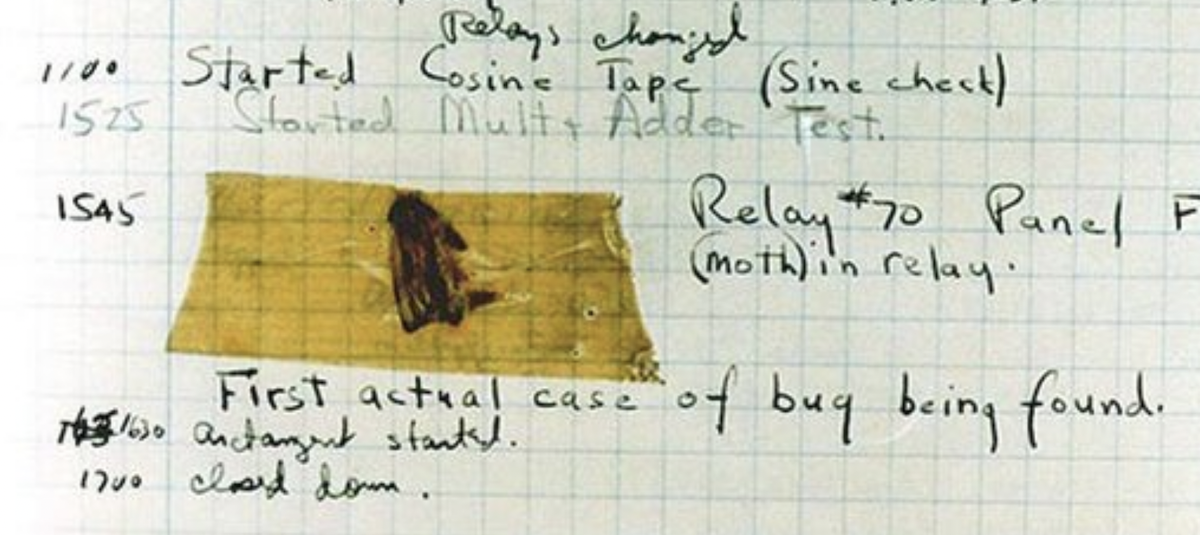• We’ll write a new program to print three “bricks”. We’ll call it buggy.c:
#include <stdio.h>

int main(void)
{
for (int i = 0; i <= 3; i++)
{
printf("#\n");
}
}

• This program compiles and runs, but we have a logical error since we see four bricks:
$make buggy$ ./buggy
#
#
#
#

• We might see the problem already, but if we didn’t, we could add another printf temporarily:
  #include <stdio.h>

int main(void)
{
for (int i = 0; i <= 3; i++)
{
printf("i is %i\n", i);
printf("#\n");
}
}

• Now, we can see that i started at 0 and continued until it was 3, but we should have our for loop stop once it’s at 3, with i < 3 instead of i <= 3:
$make buggy$ ./buggy
i is 0
#
i is 1
#
i is 2
#
i is 3
#

• In our instance of VS Code, we have another command, debug50, to help us debug programs. This is a tool that runs the debugger built into VS Code, a program that will walk through our own programs step-by-step and let us look at variables and other information while our program is running.
• First, we’ll click next to line 5 in our program to make a red dot appear: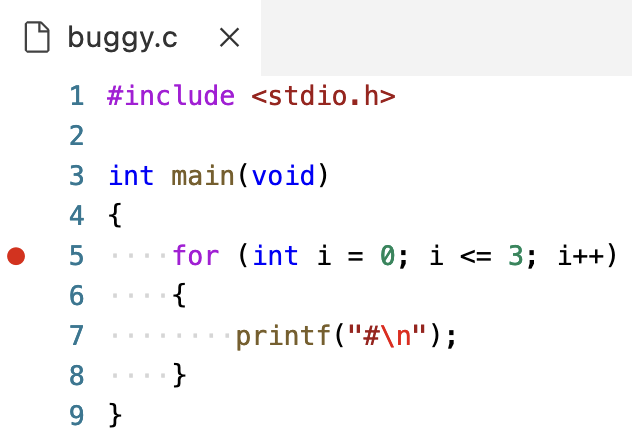• Then, we’ll run the command debug50 ./buggy, which might open a tab called Debug Console. We should go back to the tab labeled Terminal, so we can see our program’s output still: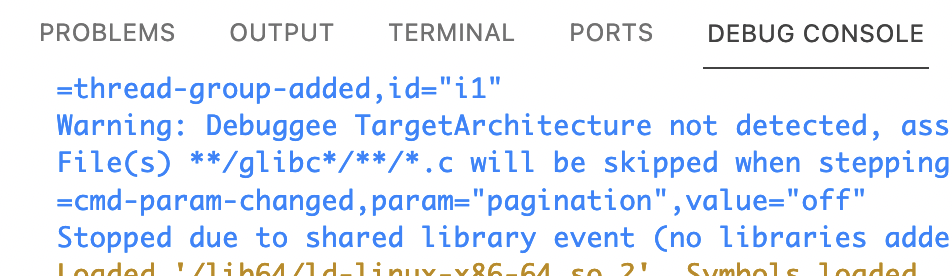• The red dot we added was a breakpoint, a place where we want our debugger to pause our program’s execution. Line 5 is now highlighted in yellow since it hasn’t executed yet: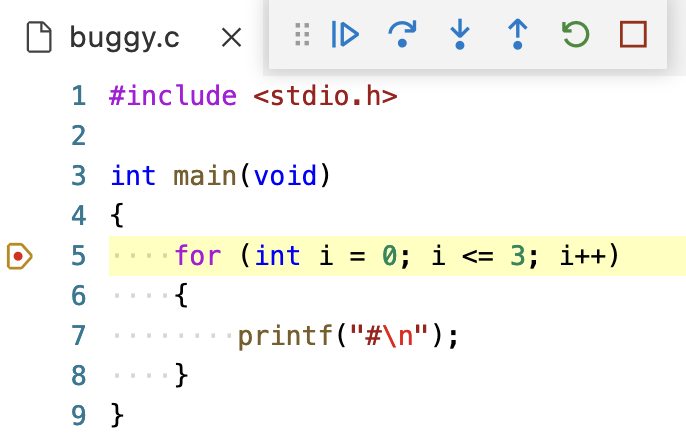• The buttons that have appeared will allow us to control our program. We can hover over each of them to see their labels. The first button that looks like a play button will “continue” our program until the next breakpoint. The second button, which looks like a curved arrow, will “step over”, or run the next line. We’ll click that one: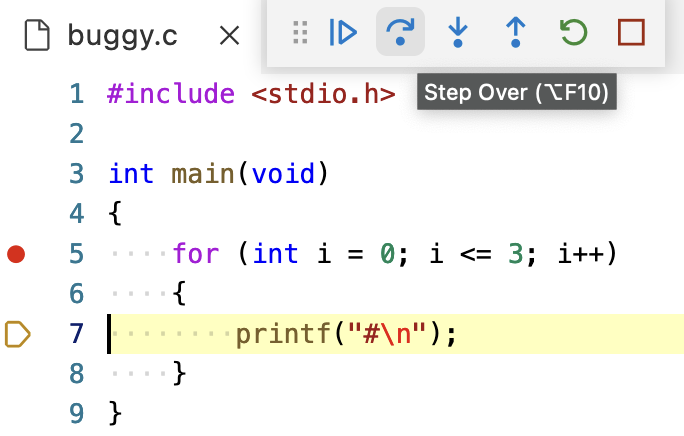• Notice that the panel on the left labeled Run and Debug has a section called Variables, where we can see their values: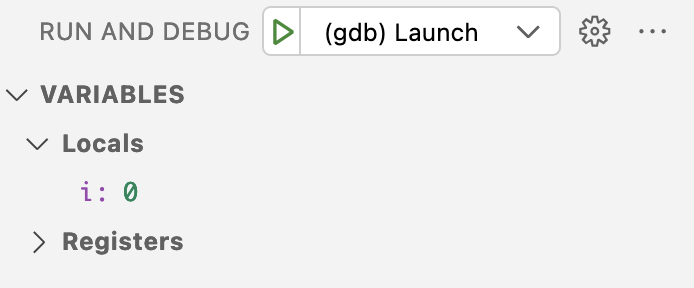• i has a value of 0.
• We’ll click the Step Over button again, and now we see a single # printed to the screen. The value of i hasn’t changed, since we’re back to line 5, but that hasn’t run yet.
• Now, we can click Step Over again, and we’ll see the value of i change to 1 as we run line 5 and move to line 7.
• We can repeat this at our pace, and see our program’s output and variables.
• To stop this process, we’ll click the rightmost button that looks like a square, and we’ll be brought back to our terminal. And we can click the red dot next to line 5 to remove the breakpoint as well.
• A third debugging technique is using a rubber duck, where we explain what we’re trying to do in our code to a rubber duck (or other inanimate object). By talking through our own code out loud step-by-step, we might realize our mistake.
• Let’s look at another buggy program:
#include <cs50.h>
#include <stdio.h>

int get_negative_int(void);

int main(void)
{
int i = get_negative_int();
printf("%i\n", i);
}

int get_negative_int(void)
{
int n;
do
{
n = get_int("Negative Integer: ");
}
while (n < 0);
return n;
}

• We’ve implemented another function, get_negative_int, to get a negative integer from the user. We’ll use a do while loop to ask the user for an integer, storing it in n, and returning it.
• We’ll include the prototype at the top of our program for our compiler.
• But when we run our program, it keeps asking us for a negative integer:
$make buggy$ ./buggy
Negative Integer: -50
Negative Integer: -5
Negative Integer: 0
0

• We could add a line to print the value:
...
int get_negative_int(void)
{
int n;
do
{
n = get_int("Negative Integer: ");
printf("n is %i\n", n);
}
while (n < 0);
return n;
}
...

• But that doesn’t help us a lot.
• We’ll set a breakpoint on line 8, int i = get_negative_int();, since it’s the first interesting line of code. We’ll run debug50 ./buggy, and click the “Step Over” button to run that line: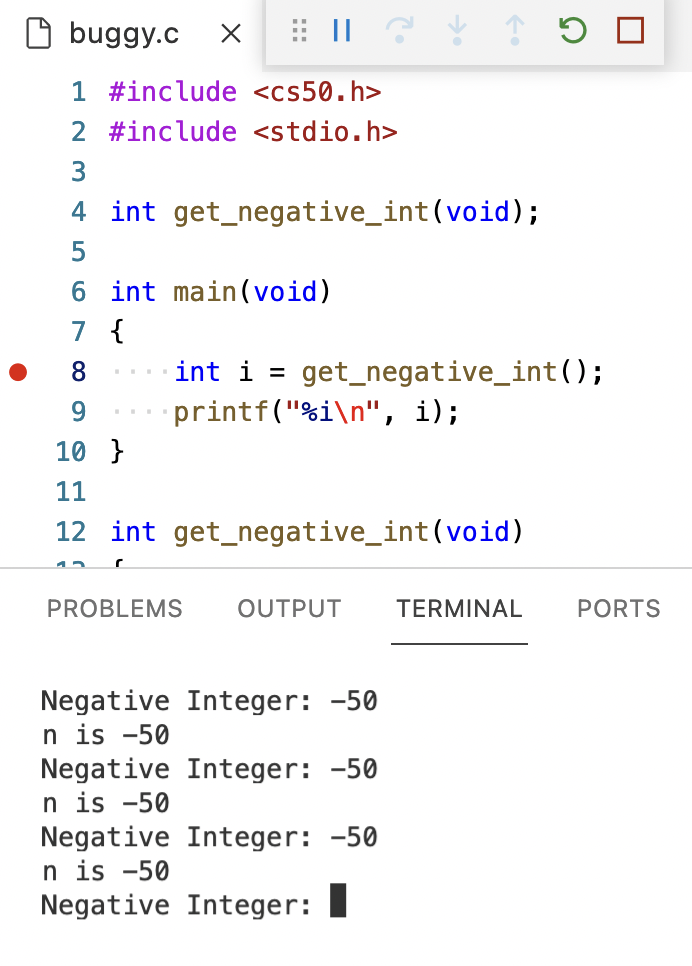• But now we are stuck in that line, and we can’t really see what it’s doing.
• We’ll stop our debugger, and start it again. This time, we’ll click the third button that looks like an arrow pointing down, “Step Into”, which will let us go into the function called on that line. Now, the line n = get_int("Negative Integer: "); within the get_negative_int function is highlighted, and hopefully we can find the mistake in our code.

## Memory

• In C, we have different types of variables we can use for storing data. Each variable is stored with a fixed number of bytes, and for most computer systems each type has the following size:
• bool, 1 byte
• A Boolean value can technically be represented with just a single bit, but for simplicity our computers use an entire byte.
• char, 1 byte
• Recall that with ASCII, we have a maximum of 256 different possible characters, since there are 8 bits in a byte.
• double, 8 bytes
• Twice as many bytes as a float.
• float, 4 bytes
• int, 4 bytes
• Recall that a 32-bit integer can represent about 4 billion different values.
• long, 8 bytes
• Twice as many bytes as an int.
• string, ? bytes
• A string takes up a variable amount of space, since it could be short or long.
• Inside our computers, we have chips called RAM, random-access memory, that stores zeroes and ones. We can think of bytes stored in RAM as though they were in a grid, one after the other: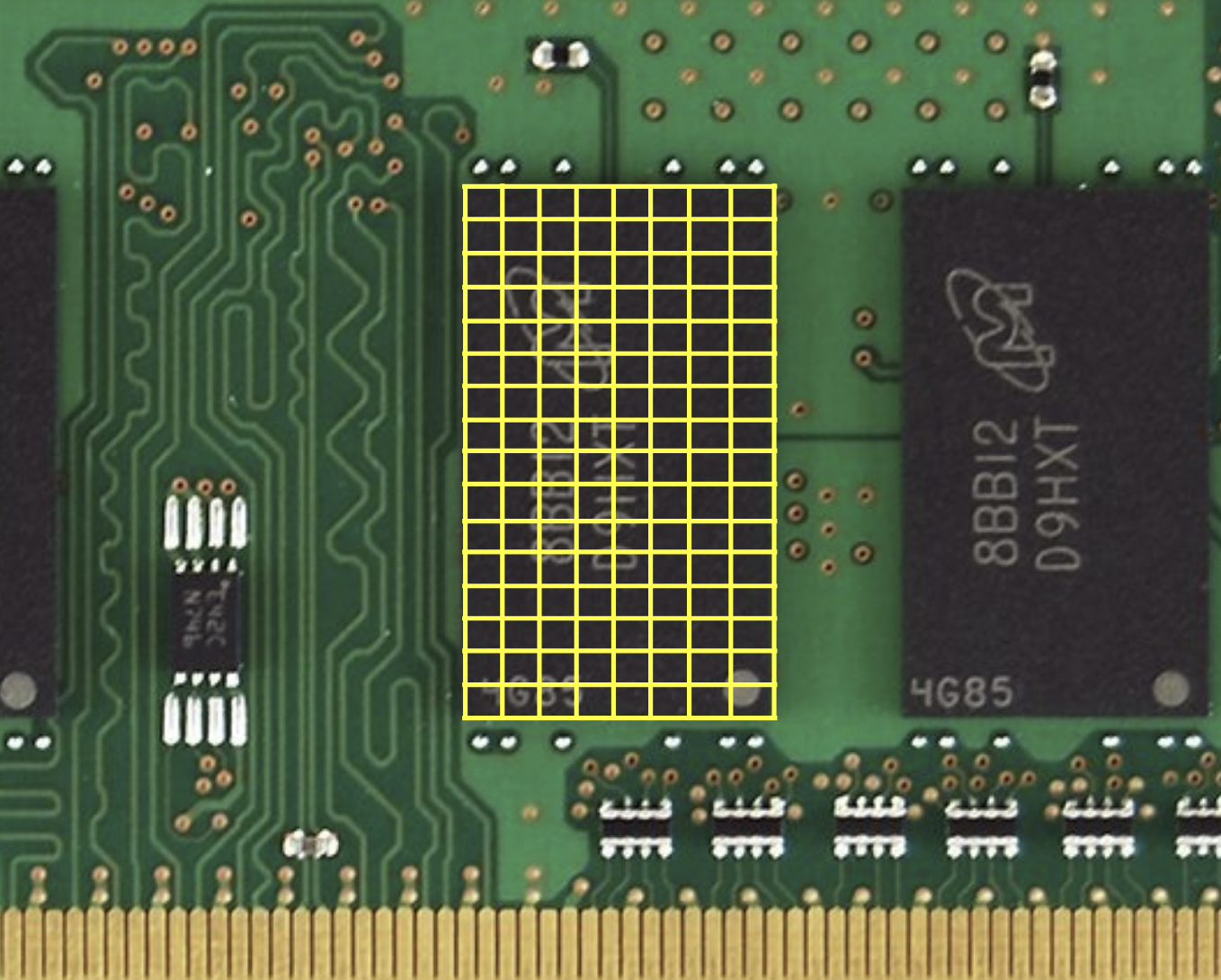• In reality, there are millions or billions of bytes per chip.
• A char which takes up one byte will take up one of those squares in RAM. An int, with 4 bytes, will take up four of those squares.
• Now, we can take for granted that bytes are stored in memory, knowing that we can work with them and build abstractions on top of them.

## Arrays

• Let’s say we wanted to take the average of three variables:
#include <stdio.h>

int main(void)
{
int score1 = 72;
int score2 = 73;
int score3 = 33;

printf("Average: %f\n", (score1 + score2 + score3) / 3);
}

• When we compile this program, we get:
$make scores scores.c:9:29: error: format specifies type 'double' but the argument has type 'int' [-Werror,-Wformat] printf("Average: %f\n", (score1 + score2 + score3) / 3); ~~ ^~~~~~~~~~~~~~~~~~~~~~~~~~~~~~ %d 1 error generated. make: *** [<builtin>: scores] Error 1  • It turns out that, dividing three integers by another integer will result in an integer, with the remainder getting thrown away. • We’ll divide by not 3, but 3.0 so the result is treated as a float: ... printf("Average: %f\n", (score1 + score2 + score3) / 3.0); ...  $ make scores
$./scores Average: 59.333333  • The design of our program isn’t ideal, since we have just three variables, and we’d have to define more and more variables. • While our program is running, the three int variables are stored in memory: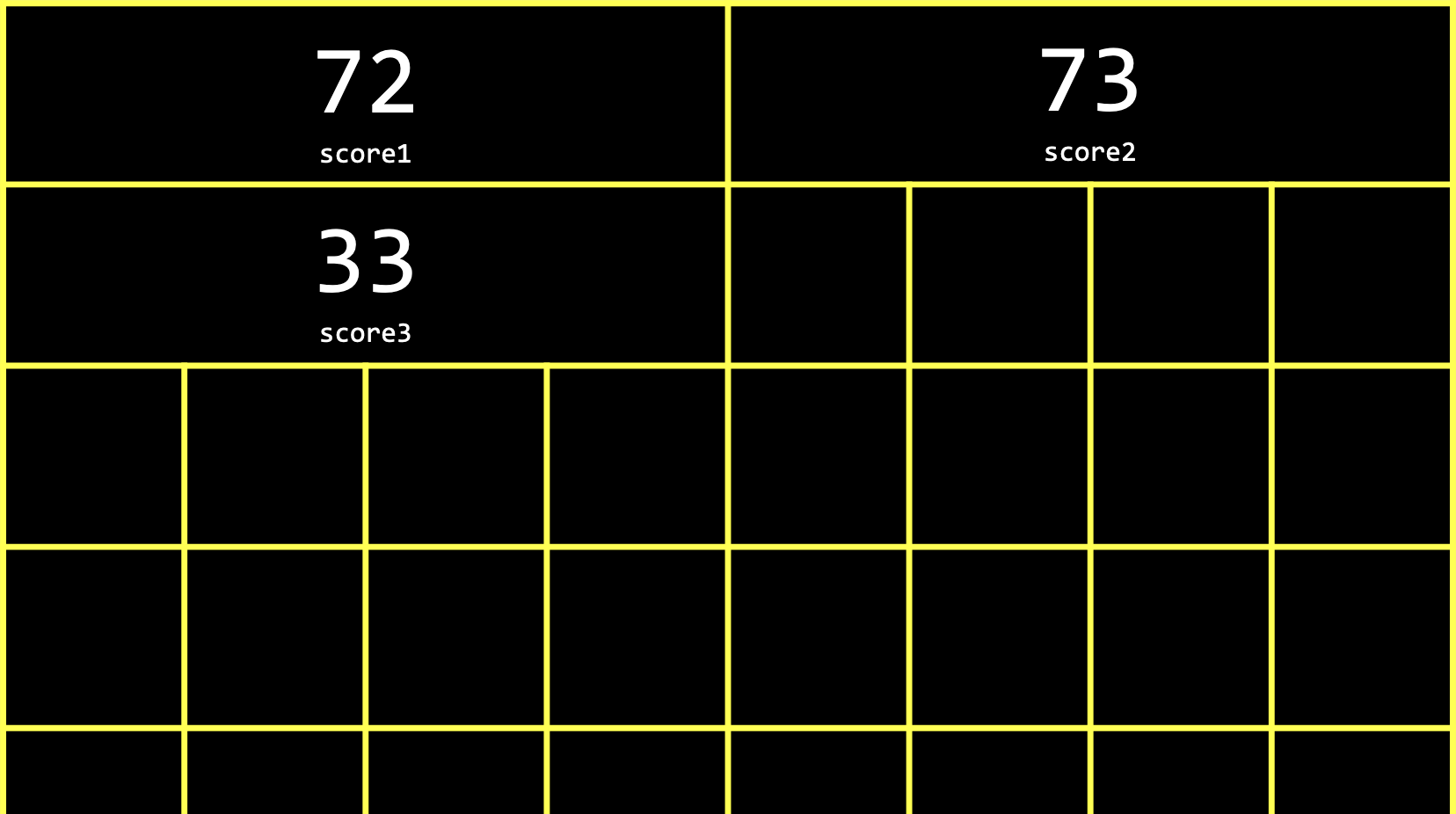• Each int takes up four boxes, representing four bytes, and each byte in turn is made up of eight bits, 0s and 1s: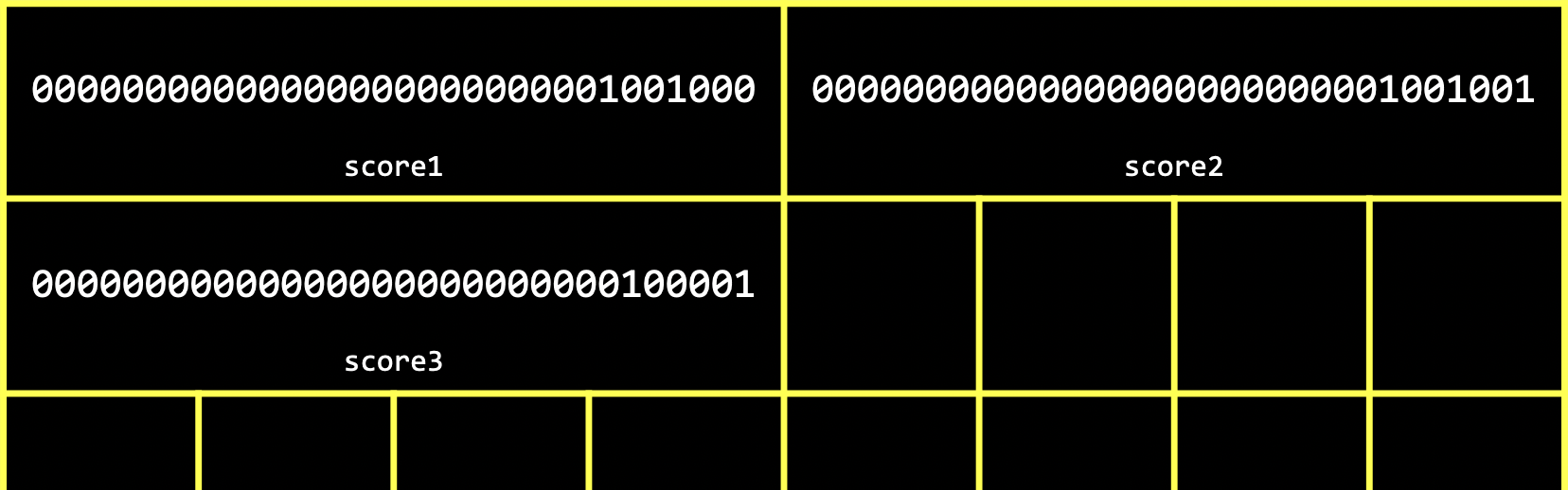• It turns out we can refer to multiple variables with one name with another type called an array. With an array, we can store values of the same type, back-to-back, or contiguously. • For example, in our program above, we can use int scores; to declare an array of three integers instead. The square brackets, , indicates how many values we want to store, and int indicates the type of each value. We also name our array scores to indicate that it stores multiple scores. • And we can assign and use variables in an array with scores = 72. With the brackets, we’re indexing into, or going to, the “0th” position in the array. Arrays are zero-indexed, meaning that the first value has index 0, and the second value has index 1, and so on. • Let’s update our program to use an array: #include <cs50.h> #include <stdio.h> int main(void) { int scores; scores = 72; scores = 73; scores = 33; printf("Average: %f\n", (scores + scores + scores) / 3.0); }  • Now, we’re storing three ints in an array, and then accessing them again later to add them together. • We’ll ask the user for each score: #include <cs50.h> #include <stdio.h> int main(void) { int scores; scores = get_int("Score: "); scores = get_int("Score: "); scores = get_int("Score: "); printf("Average: %f\n", (scores + scores + scores) / 3.0); }  • The design of our program could be improved, since we see three lines that are very similar, giving off a “code smell” that indicates we could improve it somehow. Since we can set and access items in an array based on their position, and that position can also be the value of some variable, we can actually use a for loop: #include <cs50.h> #include <stdio.h> int main(void) { int scores; for (int i = 0; i < 3; i++) { scores[i] = get_int("Score: "); } printf("Average: %f\n", (scores + scores + scores) / 3.0); }  • Now, instead of setting each value, we use a for loop and use i as the index of each item in the array. • And we repeated the value 3, representing the length of our array, in two different places. So we can ask the user and use a variable, n, for the number of scores: ... int n = get_int("How many scores? "); int scores[n]; for (int i = 0; i < n; i++) { scores[i] = get_int("Score: "); } ...  ## Characters • We might have three characters we want to print: #include <stdio.h> int main(void) { char c1 = 'H'; char c2 = 'I'; char c3 = '!'; printf("%c%c%c\n", c1, c2, c3); }  $ make hi
$./hi HI!  • We can use %c to print out each character. • Let’s see what happens if we change our program to print c as an integer: #include <stdio.h> int main(void) { char c1 = 'H'; char c2 = 'I'; char c3 = '!'; printf("%i %i %i\n", c1, c2, c3); }  $ make hi
$./hi 72 73 33  • It turns out that printf can print chars as integers, since each character is really stored as an ASCII value with zeroes and ones. • We can explicitly convert chars to ints as well with: printf("%i %i %i\n", (int) c1, (int) c2, (int) c3);  • When we convert a float to an int, however, we’ll lose some information, like the values after the decimal point. ## Strings • We can see the same output as above by using a string variable: #include <cs50.h> #include <stdio.h> int main(void) { string s = "HI!"; printf("%s\n", s); }  $ make hi
$./hi HI!  • It turns out that strings are actually just arrays of characters, and defined not in C but by the CS50 library. • Since our string is called s, which is just an array, each character can be accessed with s, s, and so on: #include <cs50.h> #include <stdio.h> int main(void) { string s = "HI!"; printf("%i %i %i\n", s, s, s); }  $ make hi
$./hi 72 73 33  • In memory, our three char variables might have been stored like this: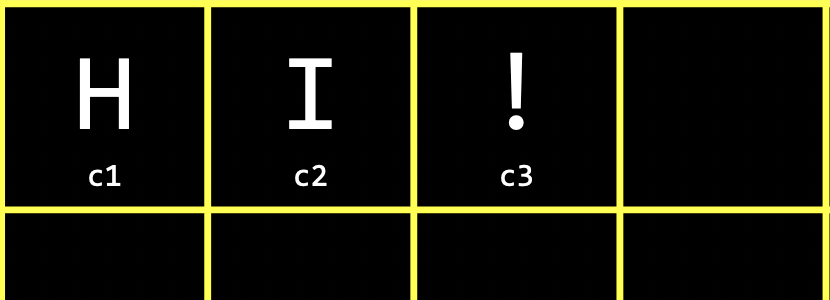• Each character in our string is stored in a byte of memory as well: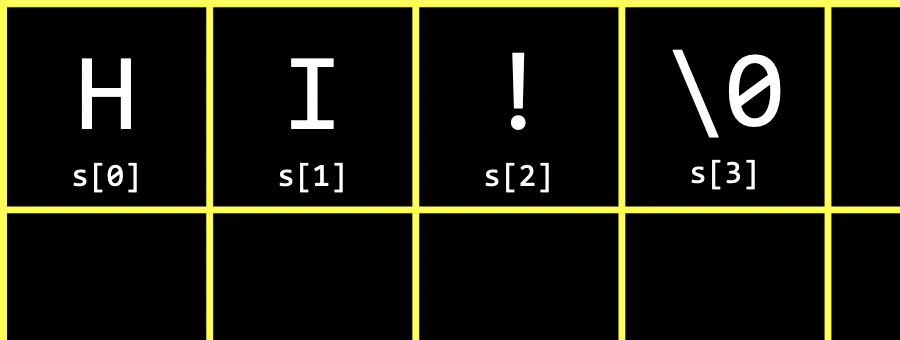• In C, strings end with a special character, '\0', or a byte with all eight bits set to 0, so our programs have a way of knowing where the string ends. This character is called the null character, or NUL. So, we actually need four bytes to store our string with three characters. • Other data types we’ve seen so far don’t need anything else at the end, since they are all set to a fixed size. Other languages or libraries might have custom types that represent numbers with a variable number of bytes as well, so there’s more precision, but that might ultimately be implemented with recording how many bytes each number is. • We can even print the last byte in our string to see that its value is indeed 0: #include <cs50.h> #include <stdio.h> int main(void) { string s = "HI!"; printf("%i %i %i %i\n", s, s, s, s); }  $ make hi
$./hi 72 73 33 0  • Let’s try taking a look at multiple strings: #include <cs50.h> #include <stdio.h> int main(void) { string s = "HI!"; string t = "BYE!"; printf("%s\n", s); printf("%s\n", t); }  $ make hi
$./hi HI! BYE!  • s takes up 4 bytes, and t takes up 5. • We can write a program to determine the length of a string: #include <cs50.h> #include <stdio.h> int main(void) { string name = get_string("Name: "); int i = 0; while (name[i] != '\0') { i++; } printf("%i\n", i); }  $ make length
$./length Name: HI! 3$ ./length
Name: BYE!
4
$./length Name: David 5  • We’ll get a string from the user, and use the variable i to get each character in the string. If the character isn’t \0, then we know it’s a character in the string, so we increment i. If the character is \0, then we’ve reached the end of the string and can stop the loop. Finally, we’ll print the value of i. • We can create a function to do this: #include <cs50.h> #include <stdio.h> int string_length(string s); int main(void) { string name = get_string("Name: "); int length = string_length(name); printf("%i\n", length); } int string_length(string s) { int i = 0; while (s[i] != '\0') { i++; } return i; }  • Our function, string_length, will take in a string as an argument, and return an int. • We can use a function that comes with C’s string library, strlen, to get the length of the string: #include <cs50.h> #include <stdio.h> #include <string.h> int main(void) { string name = get_string("Name: "); int length = strlen(name); printf("%i\n", length); }  • We can use CS50’s manual pages to find and learn about libraries and functions. Standard libraries come with older documentation, called manual pages, that aren’t always beginner-friendly. CS50’s version includes simpler documentation for common functions. • For example, we can search for “string” and see that strlen has a page that includes a synopsis, or summary, description, and example. • Now that we can use strlen, we can try to print each character of our string with a loop: #include <cs50.h> #include <stdio.h> #include <string.h> int main(void) { string s = get_string("Input: "); printf("Output: "); for (int i = 0; i < strlen(s); i++) { printf("%c", s[i]); } printf("\n"); }  $ make string
$./string Input: HI! Output: HI!$ ./string
Input:  BYE!
Output: BYE!

• We can print each character of s with s[i], and we can use a for loop since we know the length of the string, so we know when to stop.
• We can improve the design of this program. Our loop was a little inefficient, since we check the length of the string, after each character is printed, in our condition. But since the length of the string doesn’t change, we can check the length of the string once:
#include <cs50.h>
#include <stdio.h>
#include <string.h>

int main(void)
{
string s = get_string("Input:  ");
printf("Output: \n");
for (int i = 0, n = strlen(s); i < n; i++)
{
printf("%c\n", s[i]);
}
}

• Now, at the start of our for loop, we initialize both an i and n variable, and remember the length of our string in n. Then, we can check the values without having to call strlen to calculate the length of the string each time.
• And we did need to use a little more memory to store n, but this saves us some time with not having to check the length of the string each time.
• We can now combine what we’ve seen, to write a program that can capitalize letters:
#include <cs50.h>
#include <stdio.h>
#include <string.h>

int main(void)
{
string s = get_string("Before: ");
printf("After:  ");
for (int i = 0, n = strlen(s); i < n; i++)
{
if (s[i] >= 'a' && s[i] <= 'z')
{
printf("%c", s[i] - 32);
}
else
{
printf("%c", s[i]);
}
}
printf("\n");
}

$make uppercase$ ./uppercase
Before: hi
After:  HI
$./uppercase Before: david After: DAVID  • First, we get a string s from the user. Then, for each character in the string, if it’s lowercase (which means it has a value between that of a and z, inclusive), we convert it to uppercase. Otherwise, we just print it. • We can convert a lowercase letter to its uppercase equivalent by subtracting the difference between their ASCII values, which is 32 between a and A, and b and B, and so on. • We can search in the manual pages for “lowercase”, and it looks like there’s another library, ctype.h, that we can use: #include <cs50.h> #include <ctype.h> #include <stdio.h> #include <string.h> int main(void) { string s = get_string("Before: "); printf("After: "); for (int i = 0, n = strlen(s); i < n; i++) { if (islower(s[i])) { printf("%c", s[i] - 32); } else { printf("%c", s[i]); } } printf("\n"); }  • Based on the manual pages, islower will return a non-zero value if c, the character passed in, is lowercase. And a non-zero value is treated as true in Boolean expressions. (0 is equivalent to false.) • We can simplify even further, and just pass in each character to another function toupper, since it capitalizes lowercase characters for us, and returns non-lowercase characters as they originally were: #include <cs50.h> #include <ctype.h> #include <stdio.h> #include <string.h> int main(void) { string s = get_string("Before: "); printf("After: "); for (int i = 0, n = strlen(s); i < n; i++) { printf("%c", toupper(s[i])); } printf("\n"); }  ## Command-line arguments • Programs of our own can also take in command-line arguments, or inputs given to our program in the command we use to run it. • We can change what our main function to no longer take in void, or no arguments, and instead: #include <stdio.h> int main(int argc, string argv[]) { ... }  • argc and argv are two variables that our main function will now get automatically when our program is run from the command line. argc is the argument count, or number of arguments (words) typed in. argv[], argument vector (or argument list), is an array of the arguments (words) themselves, and there’s no size specified since we don’t know how big that will be ahead of time. • Let’s try to print arguments: #include <cs50.h> #include <stdio.h> int main(int argc, string argv[]) { printf("hello, %s\n", argv); }  $ make argv
$./argv David hello, ./argv  • The first argument, argv, is the name of our program (the first word typed, like ./hello). • We’ll change our program to print the argument we want: #include <cs50.h> #include <stdio.h> int main(int argc, string argv[]) { printf("hello, %s\n", argv); }  $ make argv
$./argv hello, (null)$ ./argv David
hello, David

• When we run our program without a second argument, we see (null) printed.
• We should make sure that we have the right number of arguments before we try to print something that isn’t there:
#include <cs50.h>
#include <stdio.h>

int main(int argc, string argv[])
{
if (argc == 2)
{
printf("hello, %s\n", argv);
}
else
{
printf("hello, world\n");
}
}

$make argv$ ./argv
hello, world
$./argv David hello, David$ ./argv David Malan
hello, world

• Now, we’ll always print something valid, though we’ll have to update our program to support more than two arguments.
• With command-line arguments, we can run our programs with input more easily and quickly.
• It turns out that our main function also returns an integer value called an exit status. By default, our main function returns 0 to indicate nothing went wrong, but we can write a program to return a different value:
#include <cs50.h>
#include <stdio.h>

int main(int argc, string argv[])
{
if (argc != 2)
{
printf("missing command-line argument\n");
return 1;
}
printf("hello, %s\n", argv);
return 0;
}

• A non-zero exit status indicates some error to the system that runs our program. Once we run return 1; our program will exit early with an exit status of 1. We might have seen error codes in the past when programs we used encountered errors as well.
• We’ll write return 0 explicitly at the end of our program here, even though we don’t technically need to since C will automatically return 0 for us.

## Applications

• We can consider phrases text and their level of readability, based on factors like how long and complicated the words and sentences are.
• For example, “One fish. Two fish. Red fish. Blue fish.” is said to have a reading level of before grade 1.
• “Mr. and Mrs. Dursley, of number four, Privet Drive, were proud to say that they were perfectly normal, thank you very much. They were the last people you’d expect to be involved in anything strange or mysterious, because they just didn’t hold with such nonsense…” might be measured to have a level of grade 7.
• Cryptography is the art of scrambling information to hide its contents. If we wanted to send a message to someone, we might want to encrypt, or somehow scramble that message so that it would be hard for others to read. The original message, or input to our algorithm, is called plaintext, and the encrypted message, or output, is called ciphertext. And the algorithm that does the scrambling is called a cipher. A cipher generally requires another input in addition to the plaintext. A key, like a number, is some other input that is kept secret: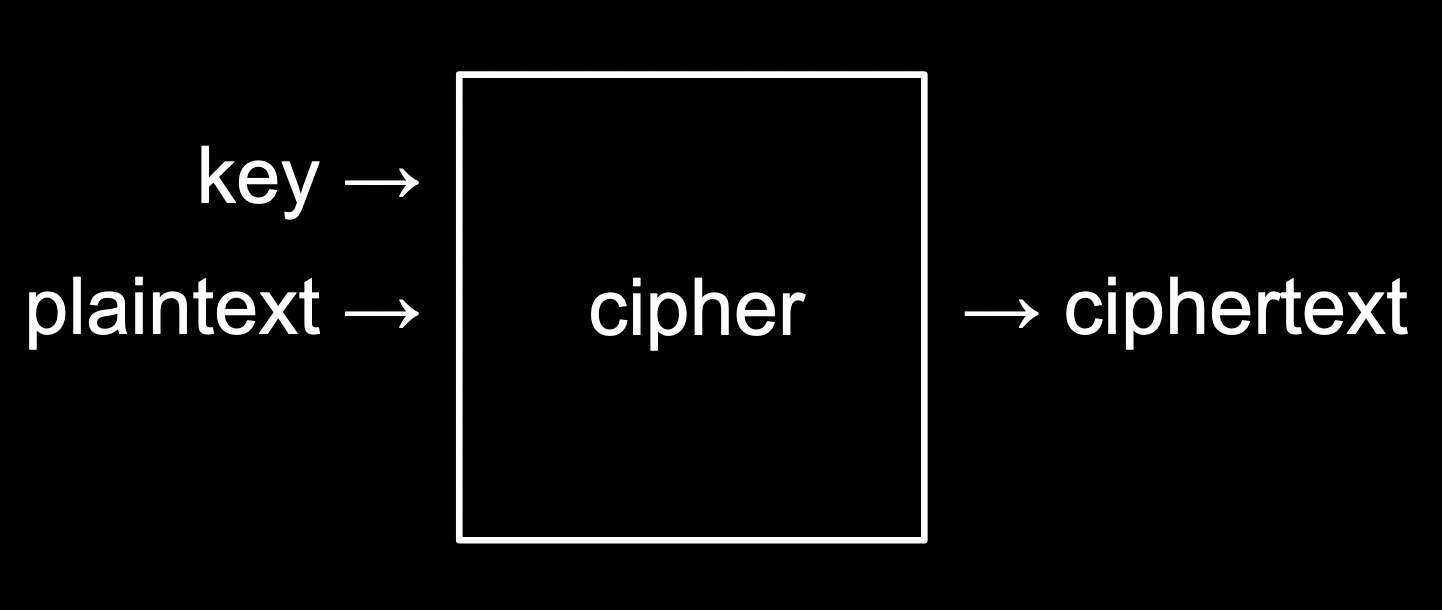• For example, if we wanted to send a message like HI! with a key of 1, we can use a simple cipher that rotates each letter forward by 1 to get IJ!.
• We could also use -1 as the key for a message of UIJT XBT DT50 to get THIS WAS CS50 as the result.